Function Repository Resource:

# SmallIntegerLinearSolve

Find a small solution to a system of linear equations over the integers

Contributed by: Daniel Lichtblau
 ResourceFunction["SmallIntegerLinearSolve"][mat,rhs] finds a small solution for x to the system of integer linear equations mat.x=rhs.

## Details and Options

The solution size is based on the Euclidean length of the resulting integer vector x.
An exactly determined system over the integers will have at most one integer-valued solution vector.
When the system is underdetermined, a "small" solution might be found from among the infinitely many solutions; this is the intended use case.
The underlying method uses lattice reduction and is not guaranteed to give the smallest possible solution.
In practice, the given solution will be close to the smallest possible solution.

## Examples

### Basic Examples (2)

Solve a linear system with integer values:

 In:=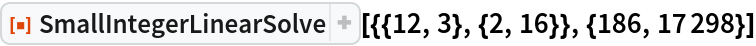Out=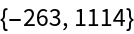Set up a random system over the integers:

 In:=Find a small integer solution:

 In:=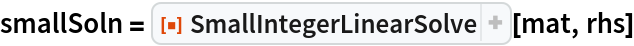Out=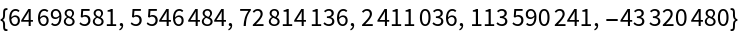Compare to the parametrized result from Solve for the corresponding system of explicit linear equations:

 In:=Out=Verify that the result of SmallIntegerLinearSolve is in the parametrized solution set:

 In:=Out=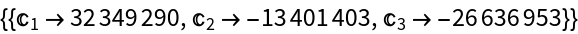### Properties and Relations (6)

Set up and solve a random system over the integers:

 In:=Out=Compare to the parametrized result from Solve for the corresponding system of explicit linear equations:

 In:=Out=Verify that the small solution is in the parametrized solution set:

 In:=Out=Though it is far slower than SmallIntegerLinearSolve, one can find a minimal solution in terms of the 1-norm (sum of absolute values):

 In:=Out=This new solution is indeed smaller in 1-norm than the small solution:

 In:=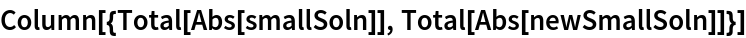Out=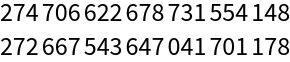However, the small solution happens to be the smaller in Euclidean norm:

 In:=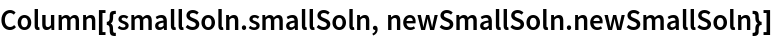Out=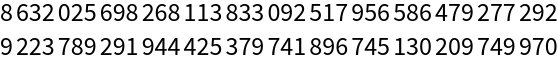### Possible Issues (1)

A system need not have an integer-valued solution:

 In:=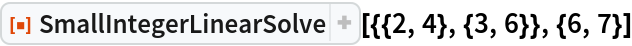Out=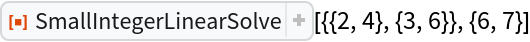## Version History

• 1.0.0 – 05 November 2019